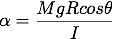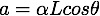# Rod falling faster than gravity

Harvard University has an interesting article on a rod whose end that falls faster than gravity around a pivot.
http://sciencedemonstrations.fas.harvard.edu/presentations/falling-faster-g
How did they derive this formula?Where R is length of rod/2, or the centre of mass.

Why did they use cos instead of sin?

Also, in this formulaWhy did they use Lcos instead of L/2 cos?

#### Attachments

The article is interesting indeed but the effect is well known for ages. Take a ##\Gamma-##shaped tube in the vertical plane such that the shoulders of this tube are vertical and horizontal. Put a chain in this tube such that half of this chain is contained in the vertical shoulder. Due to gravity the chain begins to slide and the acceleration of its links is very greater than g till a part of the chain remains in the horizontal shoulder

Last edited:
Thank you but I am still unsure about the derivation.

Why did they use cos instead of sin?

The weight of the rod has a component directed through the pivot and a tangential component causing acceleration.

•Michal Fishkin
Doc Al
MentorWhere R is length of rod/2, or the centre of mass.

Why did they use cos instead of sin?
Note that θ is the angle that the board makes with the horizontal, not the angle between the R vector and the weight. (If that was what you were thinking.)

You can also think of Rcosθ as the perpendicular distance between the line of the force (gravity) and the pivot. Or you can think of Mgcosθ as the component of the weight perpendicular to the board. (That was John Park's point.)
Also, in this formulaWhy did they use Lcos instead of L/2 cos?
They want the acceleration at the end of the rod, which has length L. (Note that R = L/2.)

•Michal Fishkin
Thanks everyone! :)
If the end of the rod was also be attached and pulling down on some mass M, would the torque also consider tension in the force?